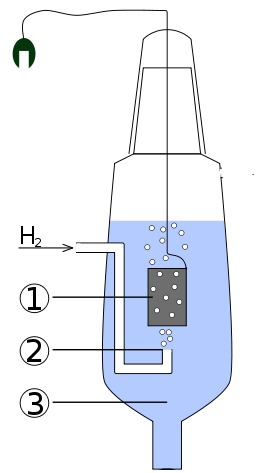Search:

# The oxidation reduction potential and the voltage of batteries

## The oxidation reduction potential

If we want to insert a redox couple the →  redox table at the right place, the procedure will work as follows: In one of the compartments we introduce oxidant and reductant (and possibly $H^+$ ions), all with molar concentrations of $1\frac{mol}{L}$ if they are soluble, otherwise in the pure state. The other compartment will be the standard hydrogen electrode:The actual electrode (1) is made of platinum which dissolves the hydrogen gas (2) constantly provided with a pressure of 1 atm, all immersed in a solution (3) of hydrogen ions with $[H^+]$ $=$ $1\frac{mol}{L}$ The voltage of this battery is then measured.

The voltage $E^o$ of a battery consisting on one side of a redox couple with molar concentrations of $1\frac{mol}{L}$, on the other side of the standard hydrogen electrode is called standard oxidation reduction potential

That is the number reported in the →   redox table. It determines the position of the couple in the table. The real potential of a redox couple depends on the concentration of the oxidant and reductant: it increases with the concentration of the oxidant (or possibly of $H^+$ ions) and decreases with the concentration of the reductant:

## The voltage of batteries

The voltage of a battery (with molar concentrations of $1\frac{mol}{L}$) is equal to the difference of the oxidation reduction potentials of its redox couples

The $Cu^{2+}1\frac{mol}{L}/Cu$ ; $Ag^+1\frac{mol}{L}/Ag$ battery has a voltage of 0,81 - 0,35 = 0,56 Volt. Its postive pole is to be found on the side of the strongest oxidant, $Ag^+$. Its negative pole on the side of the strongest reductant $Cu$